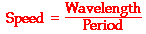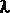# wave motion eqn can  be applid for two waves differ in considerable frequenc6y?

11 years ago

Dear Nishant,

he diagrams at the right show several "snapshots" of the production of a wave within a rope. The motion of the disturbance along the medium after every one-fourth of a period is depicted. Observe that in the time it takes from the first to the last snapshot, the hand has made one complete back-and-forth motion. A period has elapsed. Observe that during this same amount of time, the leading edge of the disturbance has moved a distance equal to one complete wavelength. So in a time of one period, the wave has moved a distance of one wavelength. Combining this information with the equation for speed (speed = distance/time), it can be said that the speed of a wave is also the wavelength/period.Since the period is the reciprocal of the frequency, the expression 1/f can be substituted into the above equation for period. Rearranging the equation yields a new equation of the form:

### Speed = Wavelength • Frequency

The above equation is known as the wave equation. It states the mathematical relationship between the speed (v) of a wave and its wavelength () and frequency (f). Using the symbolsv, and f, the equation can be rewritten as

## v = f •Cracking IIT just got more exciting,It s not just all about getting assistance from IITians, alongside Target Achievement and Rewards play an important role. ASKIITIANS has it all for you, wherein you get assistance only from IITians for your preparation and win by answering queries in the discussion forums. Reward points 5 + 15 for all those who upload their pic and download the ASKIITIANS Toolbar, just a simple  to download the toolbar….

So start the brain storming…. become a leader with Elite Expert League ASKIITIANS

Thanks

Aman Bansal